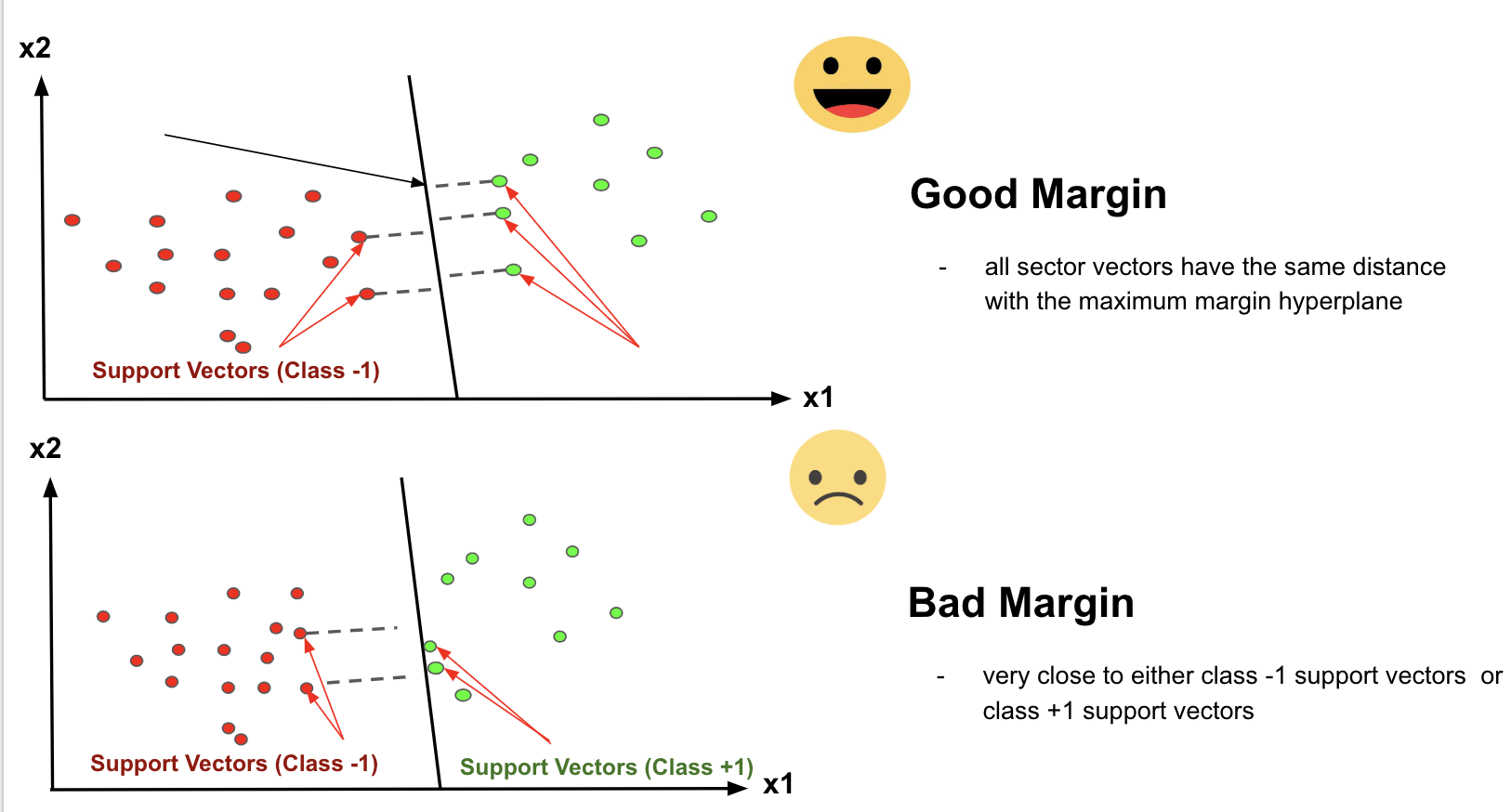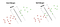# Machine Learning Quiz 03: Support Vector Machine

Let’s check your knowledge of Support Vector Machine. Here are 10 multiple-choice questions for you and there’s no time limit. Have fun!Image Source: A Top Machine Learning Algorithm Explained: Support Vector Machines (SVMs)

Question 1: What’s the objective of the support vector machine algorithm?
(A) to find an optimal hyperplane in an N-dimensional space that distinctly classifies the data points where N is the number of features.
(B) to find an optimal hyperplane in an N-dimensional space that distinctly classifies the data points where N is the number of samples.
(C) to find an optimal hyperplane in an N-dimensional space that distinctly classifies the data points where N is the number of target variables.
(D) None of these

Question 2: Support Vector Machine (SVM) can be used for _____.
(A) classification only
(B) regression only
(C) classification and regression both
(D) None of these

Question 3: In SVM, the dimension of the hyperplane depends upon which one?
(A) the number of features
(B) the number of samples
(C) the number of target variables
(D) All of the above

Question 4: In SVM, if the number of input features is 2, then the hyperplane is a _____.
(A) line
(B) circle
(C) plane
(D) None of these

Question 5: In SVM, if the number of input features is 3, then the hyperplane is a _____.
(A) line
(B) circle
(C) plane
(D) None of these

Question 6: In SVM, what is a hyperplane?
(A) decision boundaries
(B) data points
(C) features
(D) None of these

Question 7: For SVM, which options are correct? (Select two)
(A) Support vectors are data points that are closer to the hyperplane and influence the position and orientation of the hyperplane
(B) Support vectors are data points that are far away from the hyperplane and influence the position and orientation of the hyperplane
(C) Deleting the support vectors will change the position of the hyperplane
(D) Deleting the support vectors won’t change the position of the hyperplane

Question 8: In SVM, we are looking to maximize the margin between the data points and the hyperplane. The loss function that helps maximize the margin is called ______.
(A) hinge loss
(B) categorical cross-entropy loss
(C) binary cross-entropy loss
(D) None of these

Question 9: The distance of the vectors from the hyperplane is called the margin which is a separation of a line to the closest class points. We would like to choose a hyperplane that maximizes the margin between classes. Which options are true for the margin? (Select two)Image source: A Top Machine Learning Algorithm Explained: Support Vector Machines (SVMs)

(A) Hard margin — if the training data is linearly separable, we can select two parallel hyperplanes that separate the two classes of data, so that the distance between them is as large as possible.
(B) Hard margin — if the training data is linearly separable, we can select two parallel hyperplanes that separate the two classes of data, so that the distance between them is as small as possible.
(C) Soft margin — doesn’t allow some data points to stay in either incorrect side of hyperplane and between margin and correct side of the hyperplane.
(D) Soft margin — allows some data points to stay in either incorrect side of hyperplane and between margin and correct side of the hyperplane.

Question 10: Which options are true for SVM? (Select two)
(A) The distance of the vectors from the margin is called the hyperplane
(B) The loss function that helps minimize the margin is called hinge loss
(C) SVM can solve the linearly separable data points
(D) SVM can solve the data points that are not linearly separable

The solutions will be published in the next quiz Machine Learning Quiz 04. Happy learning. If you like the questions and enjoy taking the test, leave a clap for me. Feel free to discuss/share your thoughts on these questions in the comment section.

The solution of previous Machine Learning Quiz 02: Ridge, Lasso — 1(D), 2(A,B), 3(A), 4(A,B), 5(A), 6(C), 7(D), 8(D), 9(A,B), 10(D)

## More from Md Shahidullah Kawsar

Data Scientist at IDARE in the lone star state.